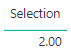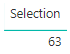Once again, I really enjoy answering questions on the Power BI Community, it keeps me challenged on how to find solutions to people’s requirements.

This time the challenge was that a user on the forum was looking to calculate the difference between 2 selections made on a slicer. In this example the slicer was on a Product.

Below is the example that I was sentI was certain that I could get this done by using a DAX measure.

I was able to get this done by using the following measure below. Not only that I created it in a way that I could select 2 products from a single slicer which would then give me the result I was looking for.

Based on the above example the result I was looking for is 63.

Below is the selection that I had on the single Product SlicerHere is the DAX measure with an explanation below.

```Selection =
VAR Top1N =
VALUE (
CALCULATE (
CONCATENATEX (
TOPN ( 1, VALUES ( 'dProduct'[Id_Product] ) ),
'dProduct'[Id_Product],
", "
)
)
)
VAR Top2N =
VALUE (
CALCULATE (
CONCATENATEX (
TOPN ( 1, VALUES ( 'dProduct'[Id_Product] ), 'dProduct'[Id_Product], DESC ),
'dProduct'[Id_Product],
", "
)
)
)
VAR FirstSelectedValue =
CALCULATE ( SUM ( 'fSales'[Quantity] ), 'dProduct'[Id_Product] = Top1N )
VAR SecondSelectedValue =
CALCULATE ( SUM ( 'fSales'[Quantity] ), 'dProduct'[Id_Product] = Top2N )
RETURN
SecondSelectedValue - FirstSelectedValue
```
• Lines 2 – 11
• The first part is to convert the result into a VALUE so that it can then be used later in the filter of the measure.
• Here I am finding the first or Top1N value from the concatenated list
• There is already a relationship between the fact table and the product table on the column ID_Product
• The result for Product 2 is the ID_Product as shown below.
•• Lines 12 – 21
• The first part is to convert the result into a VALUE so that it can then be used later in the filter of the measure.
• Here I am finding the last value from the contatenated list.
• The result for Product 4 is the ID_Product as shown below.
•• Lines 22 – 23
• Now I am taking the result from my Top1N and using this to filter my measure to return the quantity where ID_Product = 2
• As shown below is the result I am expecting
•• Lines 24 – 25
• Now I am taking the result from my Top2N and using this to filter my measure to return the quantity where ID_Product = 4
• As shown below is the result I am expecting
•• Lines 26 – 27
• I am then finally subtracting the two values to get the result I am looking for.
•UPDATE: 10 May 2019 – I got the following update from Chris, how has modified the code in a different way. What I really like is that he has put in a condition to see if more than 2 products are selected and if so then return an error. Thanks to Chris

```Selection =
VAR SelectedProducts =
VALUES ( dProduct[Id_Product] )
VAR MinValue =
MINX ( SelectedProducts, CALCULATE ( SUM ( fSales[Quantity] ) ) )
VAR MaxValue =
MAXX ( SelectedProducts, CALCULATE ( SUM ( fSales[Quantity] ) ) )
VAR Difference = MaxValue - MinValue
VAR NumProductsSelected =
COUNTROWS ( SelectedProducts )
VAR Result =
IF (
NumProductsSelected = 2,
Difference,
ERROR ( "Please select only 2 products." )
)
RETURN
Result
```

I really do love using the variables in a DAX measure, not only does it allow me to break up the measure into bite size chunks, it also allows me to debug the values to ensure that I am getting the expected results as I am working through the measure.